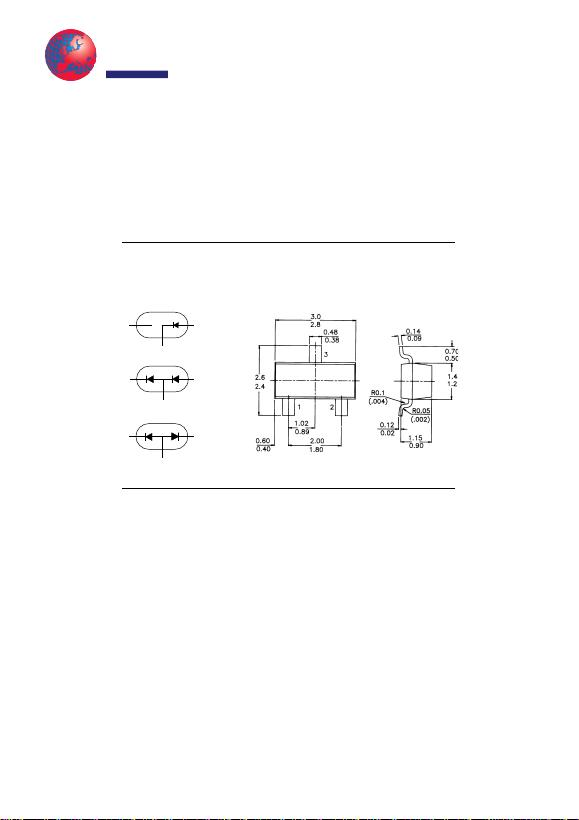SILICON PLANAR EPITAXIAL HIGH-SPEED DIODE
BAS29 single diode, BAS31 dual diodes in series and BAS35 dual diodes, common anodes.
ABSOLUTE MAXIMUM RATINGS (per diode)
Continuous reverse voltage VRmax. 90 V
Repetitive peak forward current IFRM max. 600 mA
Forward current IFmax. 250 mA
Junction temperature Tjmax. 150 °C
Forward voltage at IF = 50 mA VF<0.84 V
Reverse recovery time when switched from
IF = 30 mA to IR = 30 mA; RL = 100 ;
measured at IR = 3 mA trr <75 ns
RATINGS (per diode) (at TA = 25°C unless otherwise specified)
Limiting values
Continuous reverse voltage VRmax. 90 V
Repetitive peak forward current IFRM max. 600 mA
Repetitive peak reverse current IRRM max. 600 mA
BAS29, BAS31, BAS35
PACKAGE OUTLINE DETAILS
ALL DIMENSIONS IN mm
Marking
BAS29– L20
BAS31 – L21
BAS35 – L22
12
3
12
3
12
3
BAS29
BAS31
BAS35
SOT-23
Transys
Electronics
LI
M
ITE
DAverage rectified forward current
(averaged over any 20 ms period) IF(AV) max. 250 mA
Non-repetitive peak forward current
t = 1 µs; Tj = 25 °C prior to surge; per crystal IFSM max. 3 A
t = 1 s; Tj = 25 °C prior to surge; per crystal max. 0.75 A
Forward current (D) IFmax. 250 mA
Repetitive peak reverse energy
tp 50 µs; f 20 Hz; Tj = 25 °C ERRM
max. 5.0 mJ
Storage temperature Tstg –55 to +150 °C
Junction temperature Tj max. 150 °C
THERMAL RESISTANCE
From junction to ambient* Rth j–a = 430 K/W
CHARACTERISTICS (per diode)
Tamb = 25 °C unless otherwise specified
Forward voltage
IF = 10 mA VF<0.75 V
IF = 50 mA VF<0.84 V
IF = 100 mA VF<0.90 V
IF = 200 mA VF<1.00 V
IF = 400 mA VF<1.25 V
Reverse currents
VR = 90 V IR<100 nA
VR = 90 V; Tamb = 150 °C IR<100 µA
Reverse avalanche breakdown voltage
IR = 1 mA V(BR)R 120 to 175 V
Diode capacitance
VR = 0; f = 1 MHz Cd<35 pF
Reverse recovery time when switched from
IF = 30 mA to IR = 30 mA; RL = 100 ;
measured at IR = 3 mA trr <75ns
* When mounted on a ceramic substrate of 8 mm × 10 mm × 0.7 mm.
BAS29, BAS31, BAS35